#### How to count non blank cells?

In this article you will create the formula in Excel for non empty cells. You will learn how to count non blank cells.

## Countif function

Before doing that I will explain the basics about the COUNTIF function.

The Syntax of the COUNTIF formula is:

=COUNTIF(range, criteria)

So we have 2 arguments to be passed in this:

1. Range: It will be the range which we want to be checked for or say the range from which we will count the cells.
2. Criteria: It will be the condition which needs to be satisfied for counting the values from the range that we already specified in the range parameter.

## Example

Please find below a simple example for the same: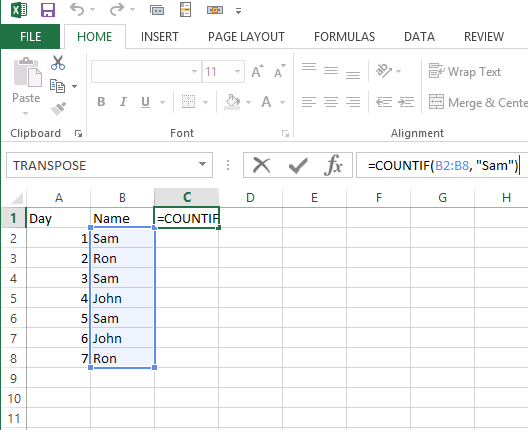In this example we have the 7 days duty chart for 3 persons.

We have to count the number of duties for a person say “Sam”

And we use the formula as below: =COUNTIF(B2:B8, “Sam”)

Here range is: B2:B8

Criteria is : “Sam”

So we the the result as: 3 (because Sam is repeated 3 times under the range)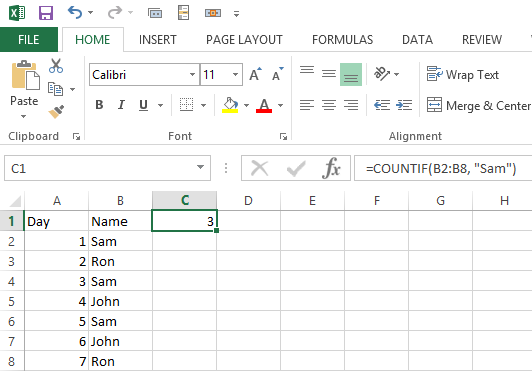We can use the same formula to check any name starting with “Sa” like:

=COUNTIF(B2:B8, “Sa*”)

Now coming to the point we need to count for non blank cells. We can use: =COUNTIF(range,”*”)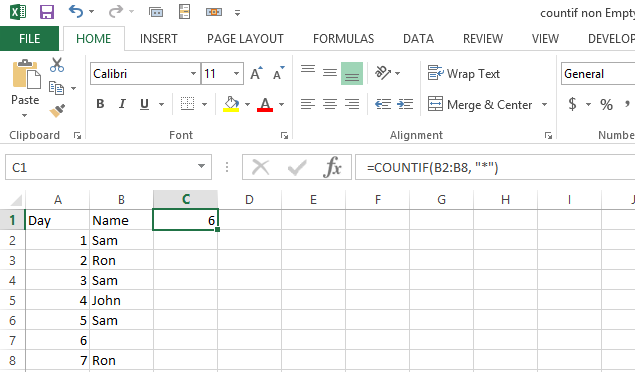As we have only 6 entries, the result is 6 which is correct. But if we use numbers instead of blank it fails because number is treated like blank.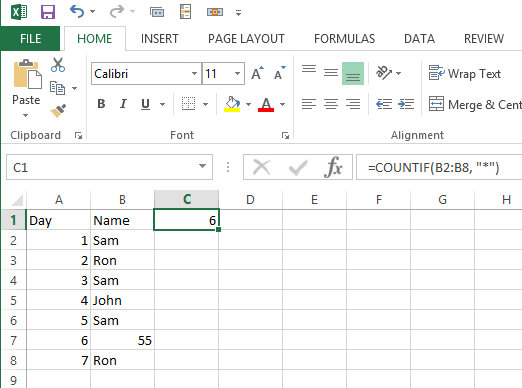## Alternative method

Another formula is: =COUNTIF(range,”<>”)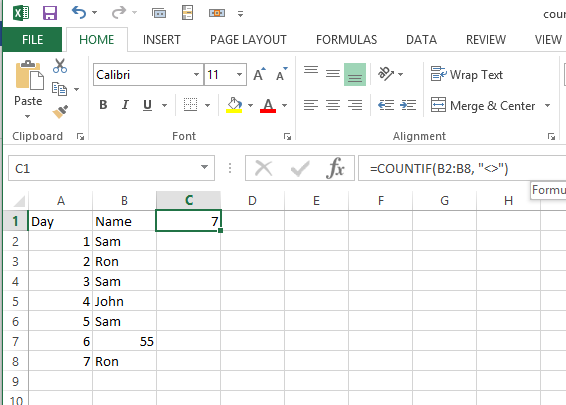Now the result is good. Number has been counted to the result of formula. This is how to count non blank cells.

## COUNTA function

Alternatively, you can also use the COUNTA function.

The COUNTA function is an Excel function that counts the number of cells in a range that are not empty or blank. It can be used to count cells that contain text, numbers, dates, or any other value, except for cells that are truly blank (i.e., cells with no content, not even spaces or formatting characters).

The syntax of the COUNTA function is as follows:

=COUNTA(value1, [value2], …)

where value1, value2, etc. are the ranges or values that you want to count. You can include up to 255 arguments in the COUNTA function.

Here are the steps:

1. Select the cell where you want to display the result.
2. Type “=COUNTA(” into the formula bar.
3. Select the range of cells that you want to count. For example, if you want to count the non-blank cells in column A, select the entire column by clicking on the column header “A”. If you want to count the non-blank cells in a specific range, select that range.
4. Close the parentheses and press Enter. The result will be the number of non-blank cells in the selected range.

Here are some examples of how to use the COUNTA function:

### Example 1: Count non-blank cells in a single column

Suppose you have a list of names in column A, and you want to count the number of non-blank cells in that column. To do this, you would enter the following formula in an empty cell:

=COUNTA(A:A)

This formula will count all the cells in column A that are not blank.

### Example 2: Count non-blank cells in multiple columns

Suppose you have a table with several columns, and you want to count the total number of non-blank cells in all the columns. To do this, you would enter the following formula in an empty cell:

=COUNTA(A1:F10)

This formula will count all the cells in the range A1:F10 that are not blank.

### Example 3: Count non-blank cells with mixed data types

Suppose you have a range of cells that contains both text and numbers, and you want to count the number of non-blank cells in that range. To do this, you would enter the following formula in an empty cell:

=COUNTA(A1:A10,B1:B10,C1:C10)

This formula will count all the cells in the ranges A1:A10, B1:B10, and C1:C10 that are not blank.

In summary, the COUNTA function is a useful tool for counting non-blank cells in Excel, and it can be used in a variety of scenarios.